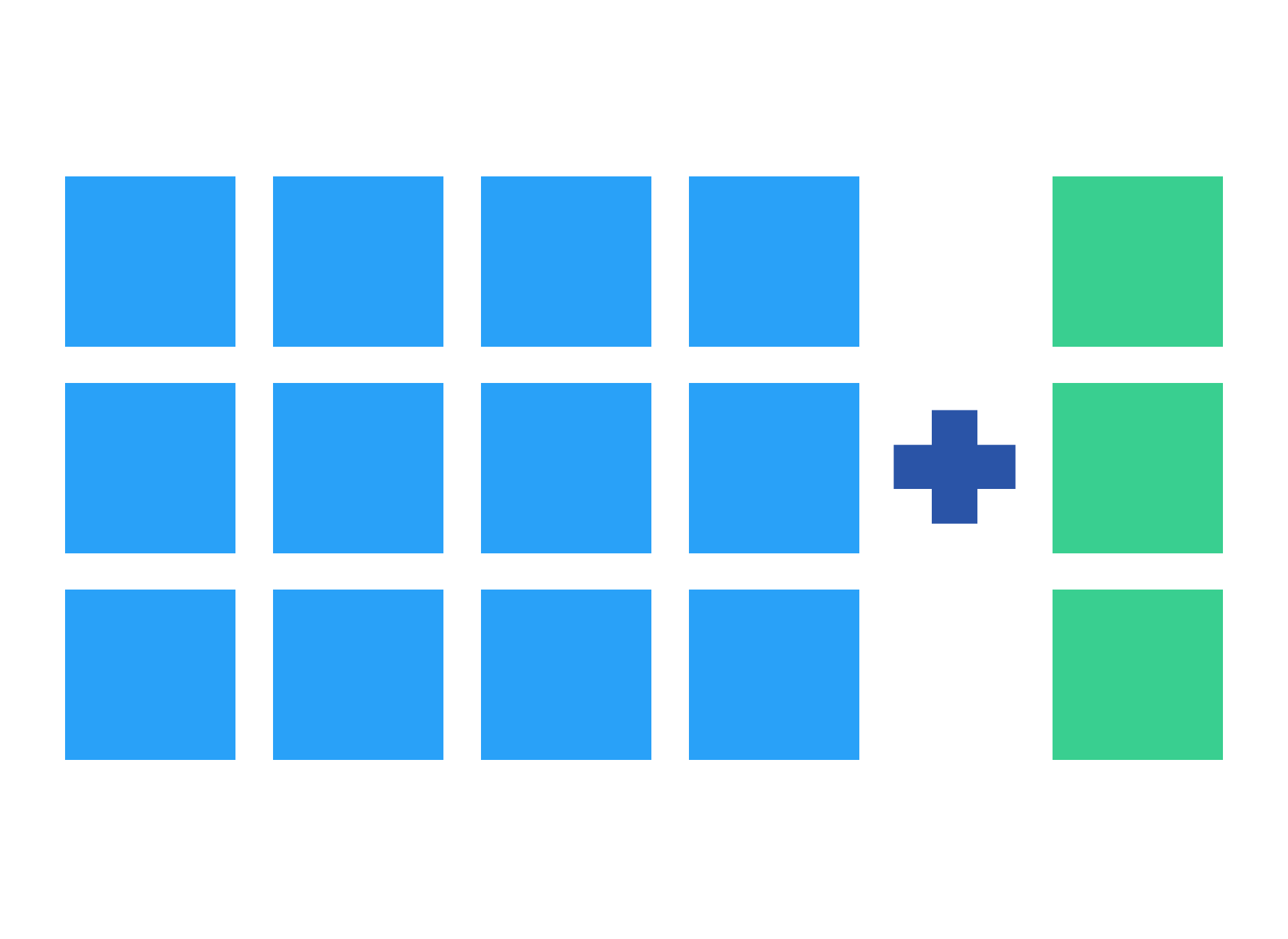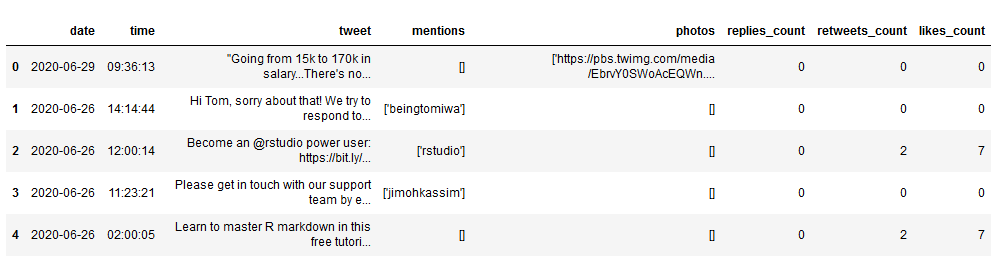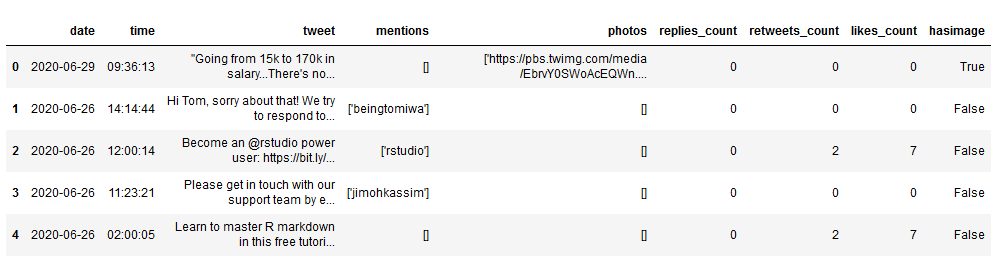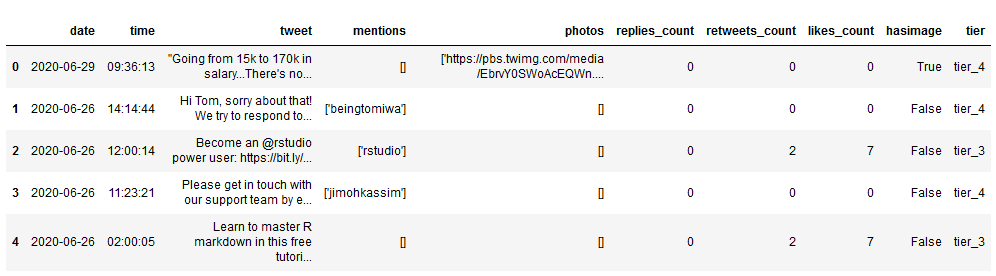July 1, 2020

# Tutorial: Add a Column to a Pandas DataFrame Based on an If-Else ConditionWhen we’re doing data analysis with Python, we might sometimes want to add a column to a pandas DataFrame based on the values in other columns of the DataFrame.

Although this sounds straightforward, it can get a bit complicated if we try to do it using an if-else conditional. Thankfully, there’s a simple, great way to do this using numpy!

To learn how to use it, let’s look at a specific data analysis question. We’ve got a dataset of more than 4,000 Dataquest tweets. Do tweets with attached images get more likes and retweets? Let’s do some analysis to find out!

We’ll start by importing pandas and numpy, and loading up our dataset to see what it looks like. (If you’re not already familiar with using pandas and numpy for data analysis, check out our interactive numpy and pandas course).

``````import pandas as pd
import numpy as npWe can see that our dataset contains a bit of information about each tweet, including:

• `date` — the date the tweet was posted
• `time` — the time of day the tweet was posted
• `tweet` — the actual text of the tweet
• `mentions` — any other twitter users mentioned in the tweet
• `photos` — the url of any images included in the tweet
• `replies_count` — the number of replies on the tweet
• `retweets_count` — the number of retweets of the tweet
• `likes_count` — the number of likes on the tweet

We can also see that the `photos` data is formatted a bit oddly.

## Adding a Pandas Column with a True/False Condition Using np.where()

For our analysis, we just want to see whether tweets with images get more interactions, so we don’t actually need the image URLs. Let’s try to create a new column called `hasimage` that will contain Boolean values — `True` if the tweet included an image and `False` if it did not.

To accomplish this, we’ll use numpy’s built-in `where()` function. This function takes three arguments in sequence: the condition we’re testing for, the value to assign to our new column if that condition is true, and the value to assign if it is false. It looks like this:

``np.where(condition, value if condition is true, value if condition is false)``

In our data, we can see that tweets without images always have the value `[]` in the `photos` column. We can use information and `np.where()` to create our new column, `hasimage`, like so:

``````df['hasimage'] = np.where(df['photos']!= '[]', True, False)Above, we can see that our new column has been appended to our data set, and it has correctly marked tweets that included images as `True` and others as `False`.

Now that we’ve got our `hasimage` column, let’s quickly make a couple of new DataFrames, one for all the image tweets and one for all of the no-image tweets. We’ll do that using a Boolean filter:

``````image_tweets = df[df['hasimage'] == True]
no_image_tweets = df[df['hasimage'] == False]``````

Now that we’ve created those, we can use built-in pandas math functions like `.mean()` to quickly compare the tweets in each DataFrame.

We’ll use `print()` statements to make the results a little easier to read. We’ll also need to remember to use `str()` to convert the result of our `.mean()` calculation into a string so that we can use it in our print statement:

``````## LIKES
print('Average likes, all tweets: ' + str(df['likes_count'].mean()))
print('Average likes, image tweets: ' + str(image_tweets['likes_count'].mean()))
print('Average likes, no image tweets: ' + str(no_image_tweets['likes_count'].mean()))
print('\n')

## RETWEETS
print('Average RTs, all tweets: ' + str(df['retweets_count'].mean()))
print('Average RTs, image tweets: ' + str(image_tweets['retweets_count'].mean()))
print('Average RTs, no image tweets: ' + str(no_image_tweets['retweets_count'].mean()))``````
``````Average likes, all tweets: 6.209759328770148
Average likes, image tweets: 14.21042471042471
Average likes, no image tweets: 5.176514584891549

Average RTs, all tweets: 1.5553102230072864
Average RTs, image tweets: 3.5386100386100385
Average RTs, no image tweets: 1.2991772625280478``````

Based on these results, it seems like including images may promote more Twitter interaction for Dataquest. Tweets with images averaged nearly three times as many likes and retweets as tweets that had no images.

## Adding a Pandas Column with More Complicated Conditions

That approach worked well, but what if we wanted to add a new column with more complex conditions — one that goes beyond True and False?

For example, to dig deeper into this question, we might want to create a few interactivity “tiers” and assess what percentage of tweets that reached each tier contained images. For simplicity’s sake, lets use Likes to measure interactivity, and separate tweets into four tiers:

• `tier_4` — 2 or fewer likes
• `tier_3` — 3-9 likes
• `tier_2` — 10-15 likes
• `tier_1` — 16+ likes

To accomplish this, we can use a function called `np.select()`. We’ll give it two arguments: a list of our conditions, and a correspding list of the value we’d like to assign to each row in our new column.

This means that the order matters: if the first condition in our `conditions` list is met, the first value in our `values` list will be assigned to our new column for that row. If the second condition is met, the second value will be assigned, et cetera.

Let’s take a look at how this looks in Python code:

``````# create a list of our conditions
conditions = [
(df['likes_count'] <= 2),
(df['likes_count'] > 2) & (df['likes_count'] <= 9),
(df['likes_count'] > 9) & (df['likes_count'] <= 15),
(df['likes_count'] > 15)
]

# create a list of the values we want to assign for each condition
values = ['tier_4', 'tier_3', 'tier_2', 'tier_1']

# create a new column and use np.select to assign values to it using our lists as arguments
df['tier'] = np.select(conditions, values)

# display updated DataFrameAwesome! We’ve created another new column that categorizes each tweet based on our (admittedly somewhat arbitrary) tier ranking system.

Now, we can use this to answer more questions about our data set. For example: what percentage of tier 1 and tier 4 tweets have images?

``````#tier 4 tweets
df[(df['tier'] == 'tier_4')]['hasimage'].value_counts(normalize=True)``````
``````False    0.948784
True     0.051216
Name: hasimage, dtype: float64``````
``````#tier 1 tweets
df[(df['tier'] == 'tier_1')]['hasimage'].value_counts(normalize=True)``````
``````False    0.836842
True     0.163158
Name: hasimage, dtype: float64``````

Here, we can see that while images seem to help, they don’t seem to be necessary for success. More than 83% of Dataquest’s “tier 1” tweets — the tweets with 15+ likes — had no image attached.

While this is a very superficial analysis, we’ve accomplished our true goal here: adding columns to pandas DataFrames based on conditional statements about values in our existing columns.

Of course, this is a task that can be accomplished in a wide variety of ways. np.where() and np.select() are just two of many potential approaches. If you’d like to learn more of this sort of thing, check out Dataquest’s interactive Numpy and Pandas course, and the other courses in the Data Scientist in Python career path.